# Unit 39 Vibrationdata SineonRandom Vibration 1 Potential SineonRandom

• Slides: 36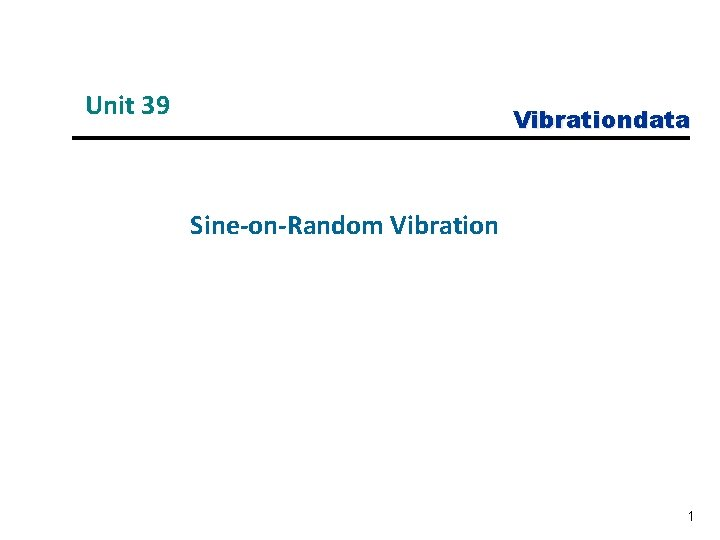Unit 39 Vibrationdata Sine-on-Random Vibration 1Potential Sine-on-Random Environments • Helicopter Vibration • Propeller-driven Aircraft • Gunfire • Launch Vehicle with Thrust Oscillation Mil-Std-810 G addresses some of these scenariosSine-on-Random Analysis and Testing Certain electronic components must be designed and tested to withstand sine-onrandom environments. The following can be done for test or analysis purposes: • Synthesize time history to satisfy sine-on- random specification • Convert sine-on-random to equivalent PSD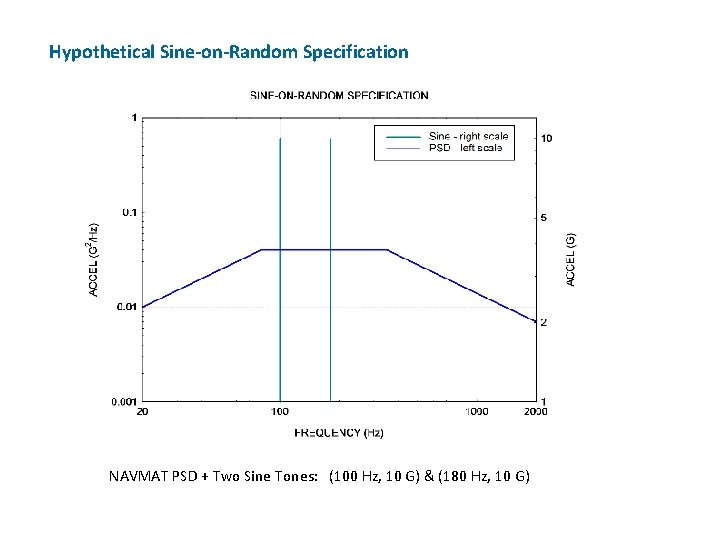Hypothetical Sine-on-Random Specification NAVMAT PSD + Two Sine Tones: (100 Hz, 10 G) & (180 Hz, 10 G)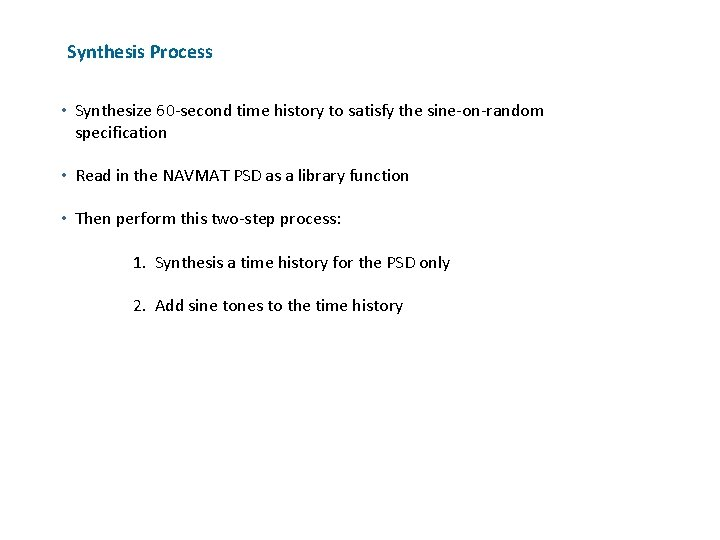Synthesis Process • Synthesize 60 -second time history to satisfy the sine-on-random specification • Read in the NAVMAT PSD as a library function • Then perform this two-step process: 1. Synthesis a time history for the PSD only 2. Add sine tones to the time history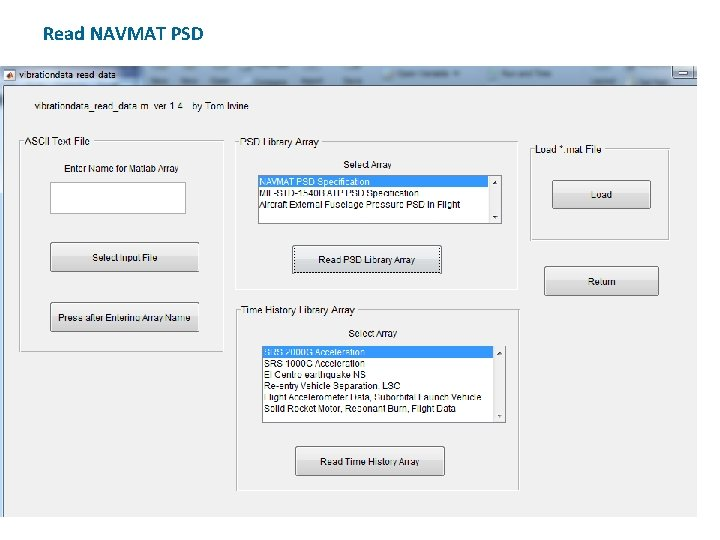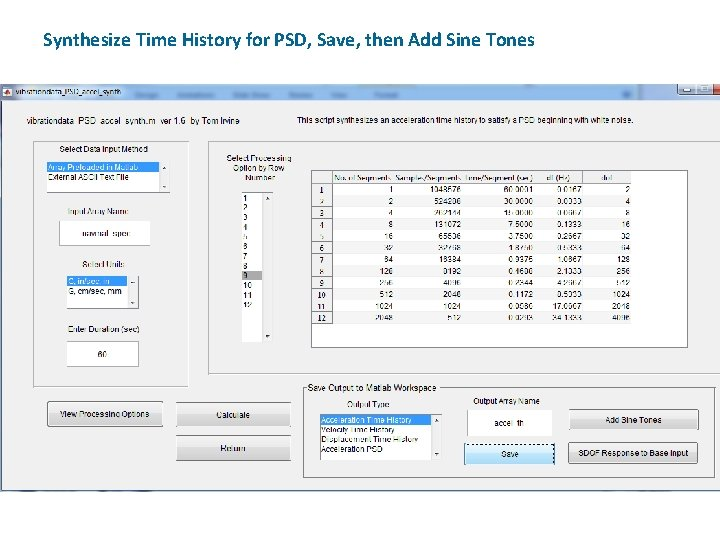Synthesize Time History for PSD, Save, then Add Sine Tones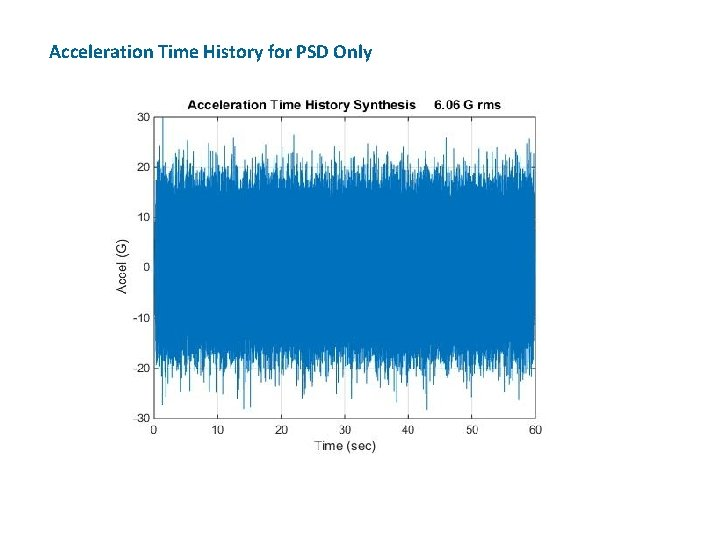Acceleration Time History for PSD OnlyAcceleration Histogram for PSD OnlyPSD Verification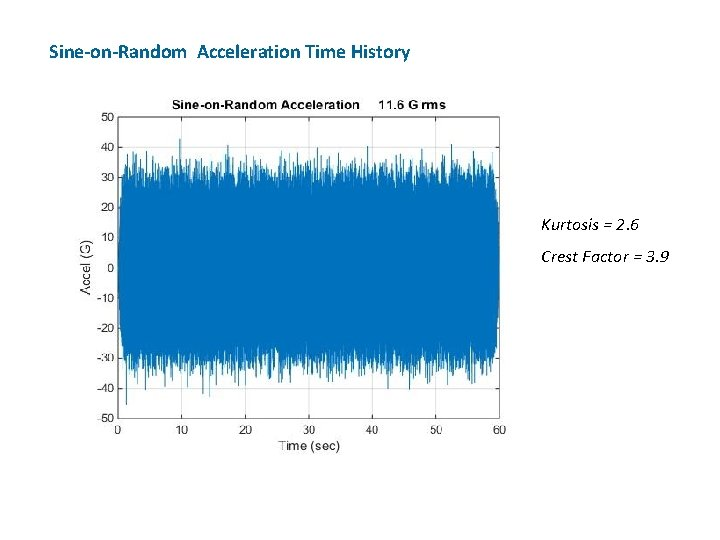Sine-on-Random Acceleration Time History Kurtosis = 2. 6 Crest Factor = 3. 9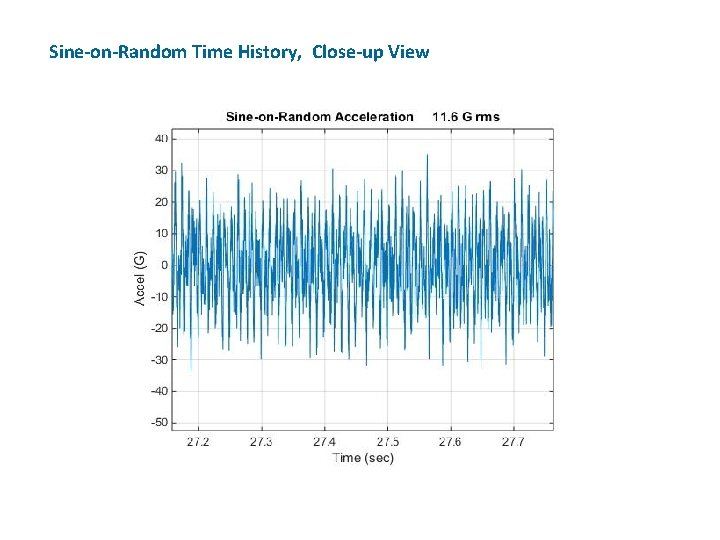Sine-on-Random Time History, Close-up ViewSine-on-Random Histogram Departs from Gaussian ideal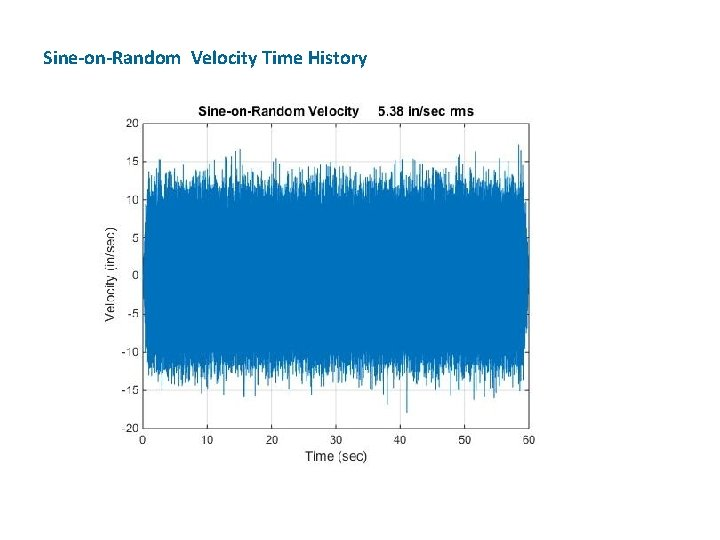Sine-on-Random Velocity Time History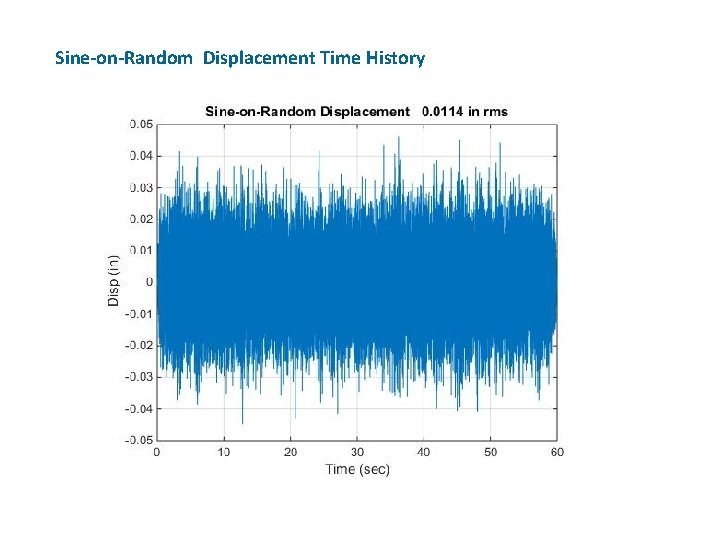Sine-on-Random Displacement Time History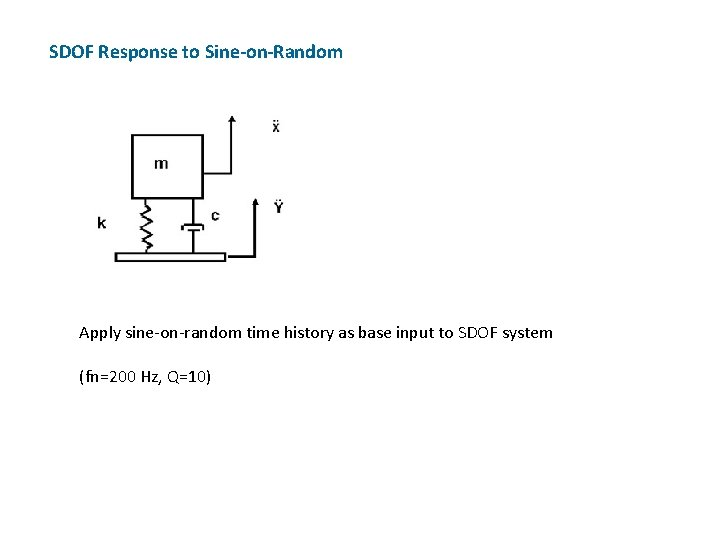SDOF Response to Sine-on-Random Apply sine-on-random time history as base input to SDOF system (fn=200 Hz, Q=10)Apply Base Excitation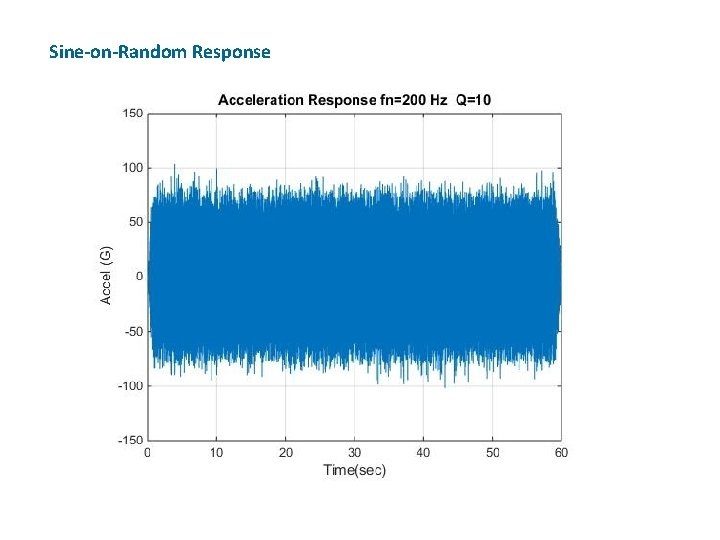Sine-on-Random Response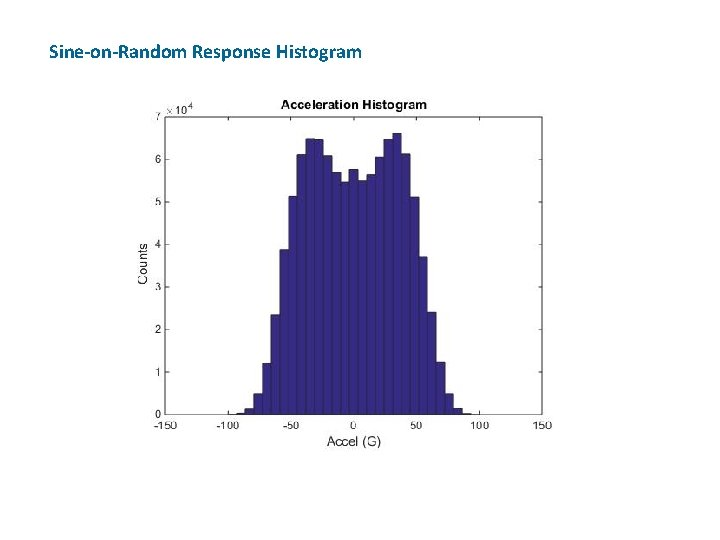Sine-on-Random Response Histogram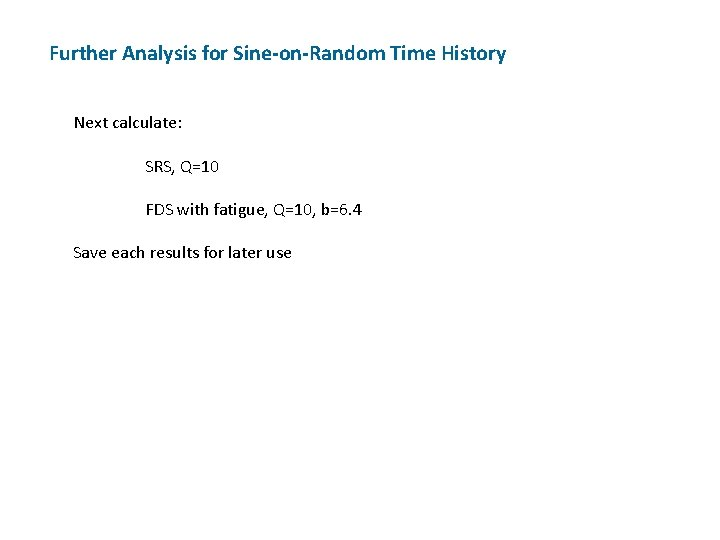Further Analysis for Sine-on-Random Time History Next calculate: SRS, Q=10 FDS with fatigue, Q=10, b=6. 4 Save each results for later useSRS CalculationFDS Calculation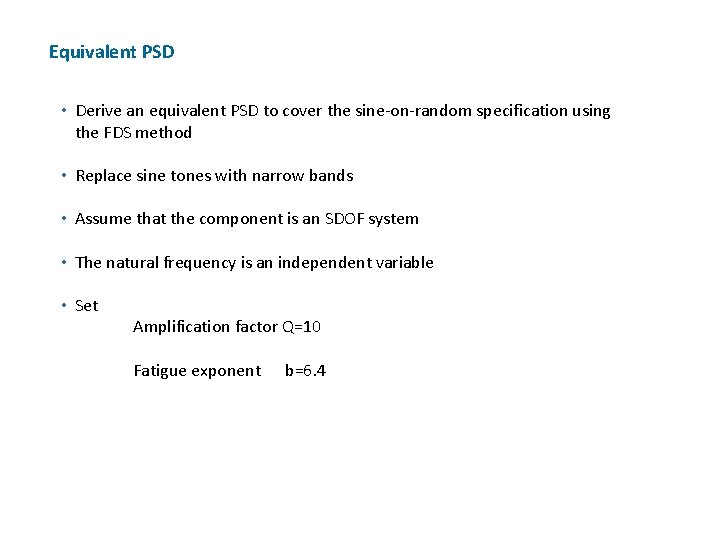Equivalent PSD • Derive an equivalent PSD to cover the sine-on-random specification using the FDS method • Replace sine tones with narrow bands • Assume that the component is an SDOF system • The natural frequency is an independent variable • Set Amplification factor Q=10 Fatigue exponent b=6. 4Conversion to PSD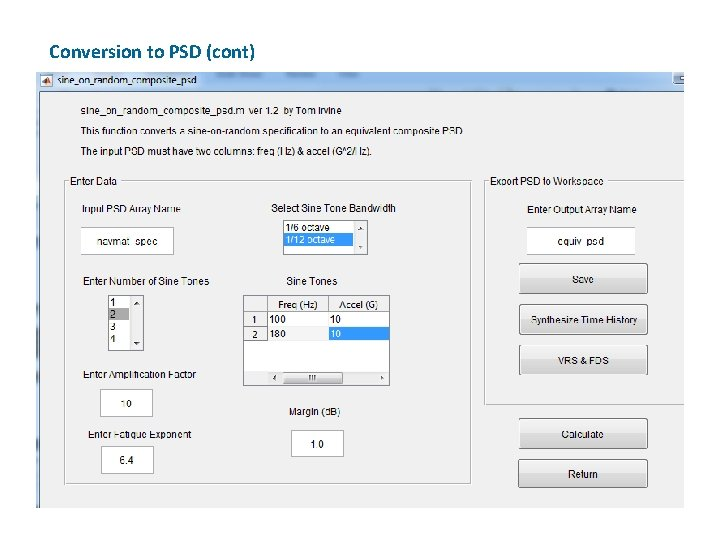Conversion to PSD (cont)Candidate Equivalent PSD Freq(Hz) Accel(G^2/Hz) 20 0. 01259 80 0. 05036 95. 76 0. 05036 97. 15 6. 342 102. 9 6. 342 104. 4 0. 05036 172. 4 0. 05036 174. 9 3. 383 185. 3 3. 383 188 0. 05036 350 0. 05036 2000 0. 008812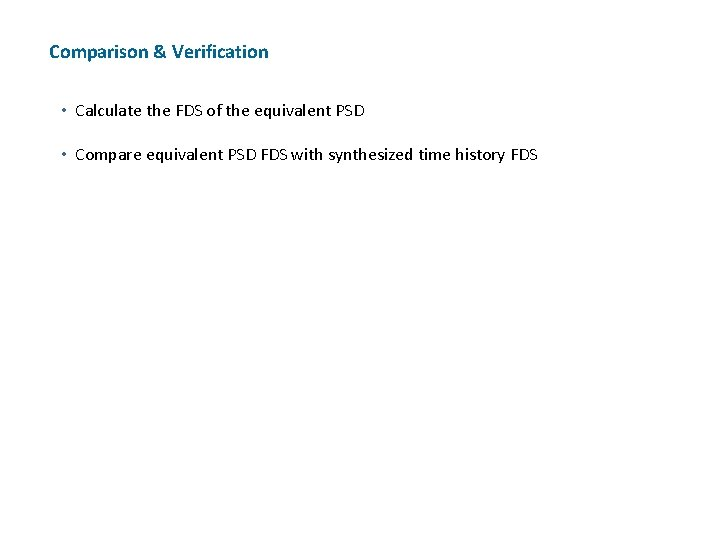Comparison & Verification • Calculate the FDS of the equivalent PSD • Compare equivalent PSD FDS with synthesized time history FDSFDS Calculation for Candidate PSD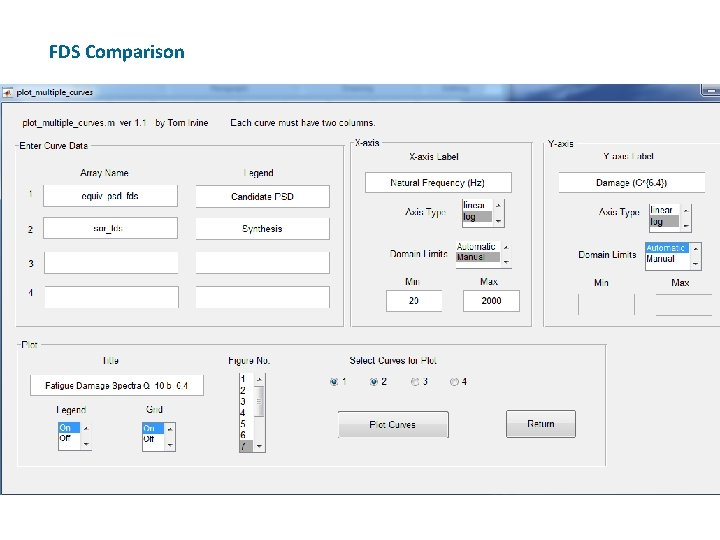FDS Comparison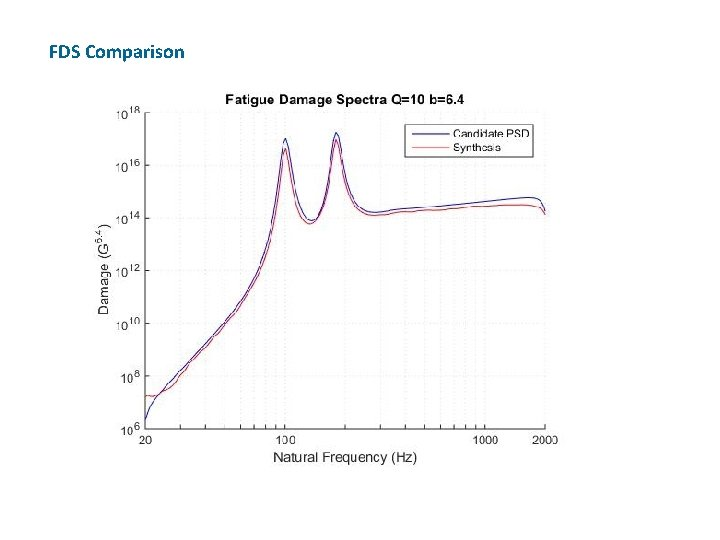FDS Comparison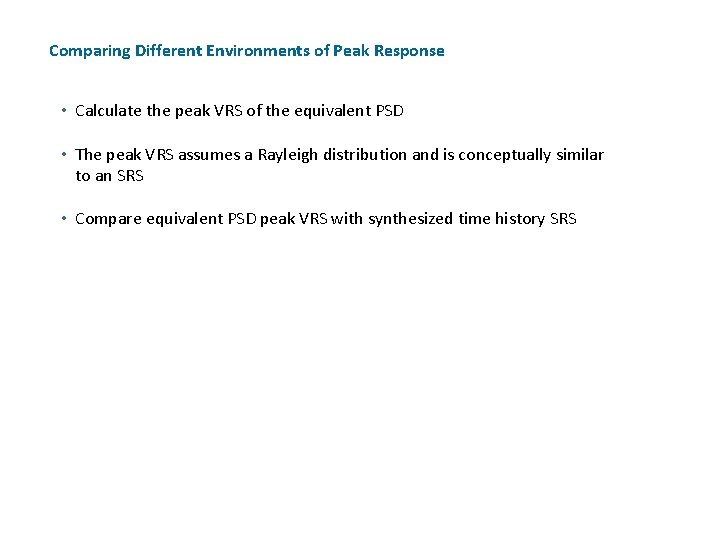Comparing Different Environments of Peak Response • Calculate the peak VRS of the equivalent PSD • The peak VRS assumes a Rayleigh distribution and is conceptually similar to an SRS • Compare equivalent PSD peak VRS with synthesized time history SRSComparing Different Environments in Terms of Damage PotentialSRS Comparison Plotting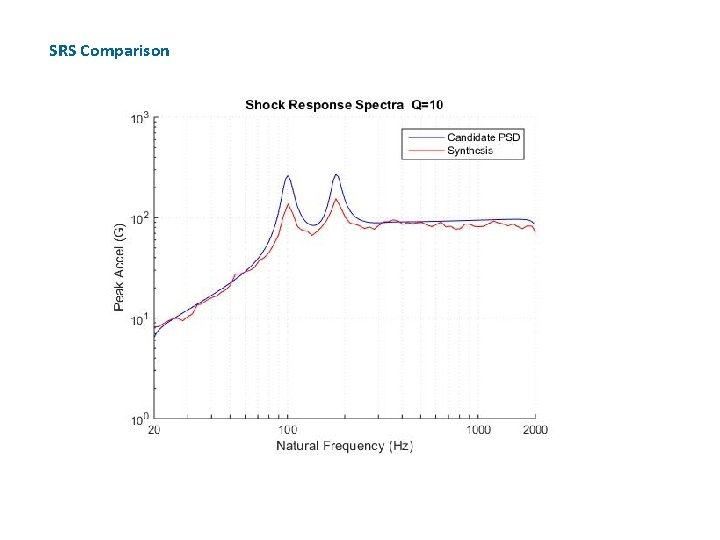SRS Comparison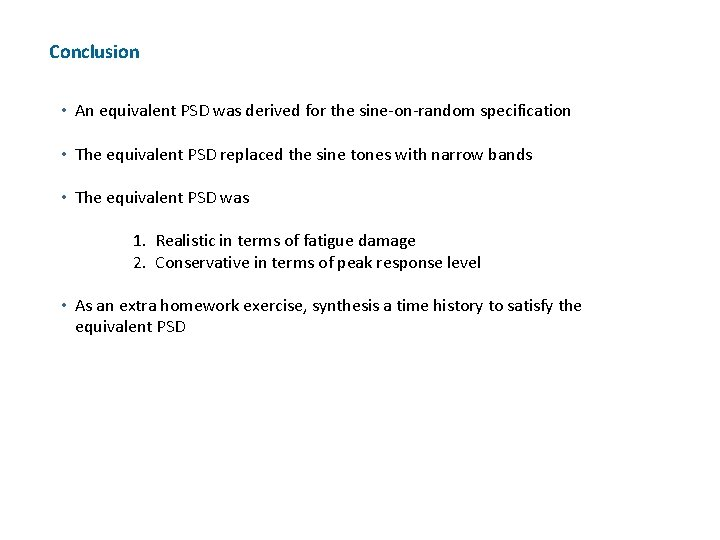Conclusion • An equivalent PSD was derived for the sine-on-random specification • The equivalent PSD replaced the sine tones with narrow bands • The equivalent PSD was 1. Realistic in terms of fatigue damage 2. Conservative in terms of peak response level • As an extra homework exercise, synthesis a time history to satisfy the equivalent PSD